# Blogging EtiquetteAcknowledge the author of the blog post.

Let the author know if you agree with him/her and why.

It is also ok to disagree with something, just let the author know why you feel that way.

One word comments are not very useful. Writing just “cool” or “nice” are not very helpful and don’t let the author of the blog post really know what you are thinking.

Always make sure you follow “netiquette”. Think if it is appropriate BEFORE you hit the submit button.

Always be polite. It does not matter if you agree or disagree with what you are reading in a blog. Don’t write anything you would be ashamed of saying to someone’s face. Don’t hurt somebody’s feelings.

# Boiling Point and States of Matter Connections and Review Assignment

Boiling Point and States of Matter Connections and Review Assignment

After watching the following video, be ready to interpret your own heating curve graph from your last blog post.

Thanks to MR. Kent’s Chemistry Page, You can find great graphs and explanations (Warning, some parts are beyond the 6th grade level expectations.)

Create a presentation that will be delivered in your next blog post describing all you have learned about the States of Matter and Boiling Point while making connections and reviewing for your upcoming assessment.

Use the Rubric HERE to make sure you have done your very best to show what you now know and understand.

HELPFUL TO THINK ABOUT AND USE AS NEEDED:

1. Demonstrations – Vacuum Chamber – marshmallow, boiling water, balloon; Ball and ring (heating it made it not go through the ring); Alcohol and Water – 50 ml + 50 ml ≠ 100 ml
2. Videos – Boiling water on Everest; Brain Pop – States of Matter  or  Matter Changing States; Eureka Videos – Solids  or Liquids ; Plasma Video
3. Readings: KS3 Bitesize; Chem4Kids Website
4. Lab Sheets
5. Blog Posts: State Change Graph: Look up what you already have done on your blog!
6. Vocabulary words –  (see below)

Don’t forget the following when presenting your work:

• Design – is it easy to read and laid out nicely
• Words – not too wordy but enough so that it is understood
• In your own words – don’t copy and paste
• Images/Media – “a picture says a 1000 words!”
• Spelling, grammar, paragraphs and all of your favorite things

Vocabulary:

• Air Pressure
• Boiling Point
• Condensation
• Diffusion
• Evaporation
• Solid
• Liquid
• Gas
• Matter
• Molecule
• Pure Substance
• State
• Vapour
• Particles
• Heating Curve
• Sublimation

# Math Summative Assessment Review for Representing and Converting Portions, Fraction Multiplication, and Integer Addition and Representation on a Number Line

Here are practice problems and answers(at the end of the post) for our upcoming assessment that is scheduled for December 10th. Please take your time to go through the different sections and practice leading up to the assessment and NOT just the night before. If you have any questions, make sure to ask me during class, at break, at lunch or at homework club time on Mondays.

### Representations of Portions: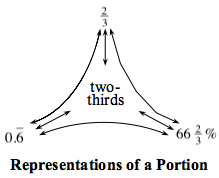• CL 2-130. Convert the following fractions to decimals and the decimals to fractions.
1.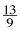2.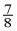3. 0.204
4. 0.47
• 2-18. Complete the Representations of a Portion Web for each number below. An example for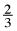is shown above in the portion web.
1.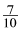2. 0.75
3. Three-fifths
4.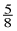### Multiplication of Fractions: Diagramming, Understanding what a Diagram Represents by figuring an equation, getting final values of multiplication of fractions

• 5-10. PARTS OF PARTS: Part One
• Representing a portion of another portion can be thought of as finding a “part of a part.”  For each of the parts of parts described below, work with your team to figure out what part of the whole is described.  For each problem, show at least one picture or diagram that helps you make sense of the problem.
1.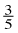of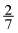2.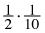• 5-14. Find each of the parts of parts described below.  For each one, create a diagram that demonstrates your thinking.  Homework Help ✎
1.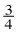of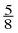2.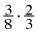3.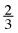of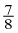4.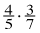• 2-89. For each of the four diagrams below, write an equation that represents the darkly shaded portion and a sentence that describes that section.
•  Example: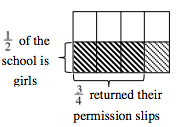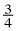of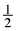is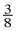•of the students at the school are girls who returned their permission slips.
•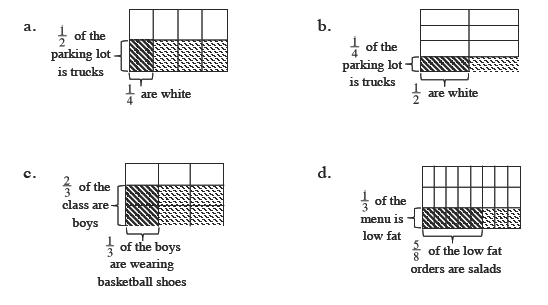• 2-90. Frieda Friendly works for a local car dealership.  She noticed thatof the cars are sedans and that half of them are white.  What fraction of the dealership’s cars are white sedans? Homework Help ✎
1. Use a unit rectangle to represent this situation.  Label the parts carefully.
2. Write an equation or a sentence that describes the situation, and answer the question.
3. Write your answer from part (b) as a decimal and as a percent.
•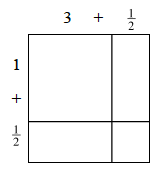• 2-97. Ronna is making a small flowerbed that is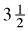feet by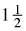feet.  She needs to find the area so she can add the correct amount of fertilizer to the soil.
• On your paper, draw the rectangle shown above.  Then complete parts (a) through (c) below.
1. Write the area in each of the four parts of the drawing.  Then find the total area.
2. Write an equation that represents the sum of the four areas.
3. What multiplication problem could you use to solve this problem?
• 2-98. Using the process shown in the previous problem, find the area of Ronna’s flowerbed if the dimensions arefeet by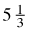feet.  Clearly label all dimensions.  Write the multiplication problem that can be used to solve this problem.

## Integers and the Number Line:

• 2-37. Below are some new distances with given lengths to help Cecil cross the tightrope.  Find at least two ways to get Cecil across.  Write your solutions as numerical expressions. 2-37a HW eTool (CPM).  2-37b HW eTool (CPM). Homework Help ✎
1. Span of tightrope:  6 feet       Given lengths:  1, 3, 4 feet
2. Span of tightrope:  8 feet       Given lengths:  2, 5, 7 feet
• 2-51.  Write an expression to represent each of the following sketches.  Then give the value of each sketch.  Homework Help ✎

1.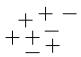2.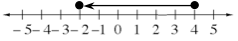3.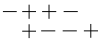4.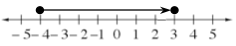• 2-58. For each expression below:
• Build each expression with + and – tiles
• Sketch each collection of + and – tiles and find the value of the expression.
• Write the solution as an equation.
• Explore using 2-58 Student eTool (CPM).
•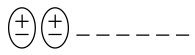For example, in part (a) below, you might draw the figure at right and record −8 + 2 = −.6
1. −8 + 2
2. −5 +(−3)
3. 2 + (−4)
4. −7 + (−7)
5. −4 + 3
6. −4 + 8 + (−2)
7. −3 + 5 + 3
8. −6 + 6

#### CL 2-130.

1.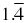2. 0.875
3.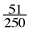4.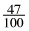2-18. See below:

1. seven-tenths, 0.7, 70%
2. seventy-five hundredths,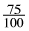=, 75%
3.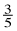= 0.6, 60%
4. five-eighths, 0.625, 62.5%
• 5-10. See below:
1.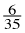2.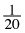or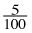• 5-14.  Diagrams will vary:
1.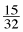2.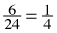3.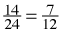4.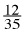• 2-89. See below:
1.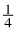of=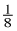of the vehicles in the parking lot are white trucks.
2.of=of the vehicles in the parking lot are white trucks.
3.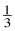of=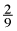of the class are boys wearing basketball shoes.
1.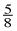of=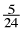of the menu is low-fat salads.
• 2-90. See below:
1. See diagram below.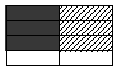2.ofof all the cars are white sedans, or·=3. 0.375, 37.5%
• 2-97.   See below:
1. See diagram below.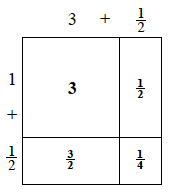2. 3 ++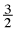+= 53. 3·  1• 2-98. Sample solution shown below. 5·  1= 8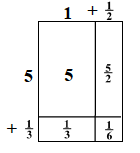• 2-37. See below:
1. Possible solutions:  4 + 1 + 1, 3 + 3, 3 + 1 + 1 + 1
2. Possible solutions:  5 + 5 + (−2), 7 + 5 + (−2) + (−2)
• 2-51.  Sample expressions:
1. 5 + (−3) = 2
2. 4 + (−6) = −2
3. −4 + 4 = 0
4. −4 + 7 = 3
• 2-58. See below:
1. −6
2. −8
3. −2
4. −14
5. −1
6. 2
7. 5
8. 0

# Density Graph test 2

Density Graph# Testing Graph post